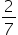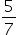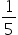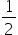Mathematics
Easy

Question

# In a lottery there are 10 prizes and 25 blanks. The probability of getting prize in a single draw is

##Hint:

## The correct answer is:### Here, it is given that in a lottery, there are 10 prizes and 25 blanks. We have to find the probability of winning a prize.Total number of outcomes = Number of prizes + Number of blanks= 10 + 25= 35Number of favourable outcomes = Number of prizes = 10Therefore, P ( getting a proze ) = 10/35= 2/7Hence, the correct option is (a).

0 ≤ P (E) ≤ 1#### With Turito Foundation.#### Get an Expert Advice From Turito.